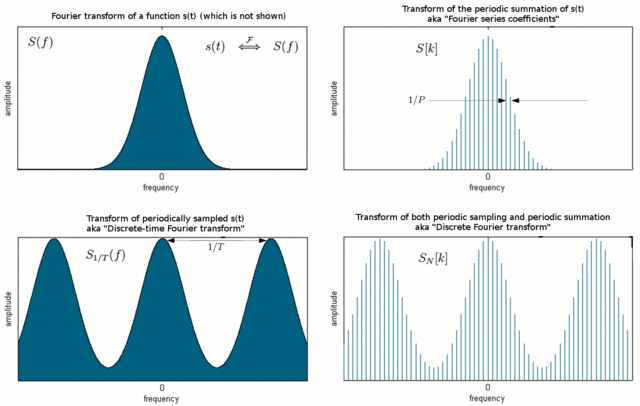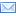#Difference Between Similar Terms and Objects

# Difference Between FFT and DFT

Fast Fourier Transform (FFT) Vs. Discrete Fourier Transform (DFT)

Technology and science go hand in hand. And there is no better example of this than digital signal processing (DSP). Digital Signal Processing is the process for optimizing the accuracy and efficiency of digital communications. Everything is data – whether it’s the images from outer space probes or seismic vibrations and anything in between. To convert these data into human readable format using computers is digital signal processing. It is one of the most powerful technologies that combines both mathematical theory and physical implementation. The study of DSP started as a graduate-level course in electrical engineering, but over time, it has become a potential gamechanger in the field of science and engineering. Suffice to say, without DSP, engineers and scientists might cease to exist.

Fourier transform is a means of mapping a signal, in the time or space domain into its spectrum in the frequency domain. The time and frequency domains are just alternative ways of representing signals and the Fourier transform is the mathematical relationship between the two representations. A change of signal in one domain would also affect the signal in the other domain, but not necessarily in the same way. Discrete Fourier Transform (DFT) is a transform like Fourier transform used with digitized signals. As the name suggests, it is the discrete version of the FT that views both the time domain and frequency domain as periodic. Fast Fourier Transform (FFT) is just an algorithm for fast and efficient computation of the DFT.## Discrete Fourier Transform (DFT)

The Discrete Fourier Transform (DFT) is one of the most important tools in digital signal processing that calculates the spectrum of a finite-duration signal. It is very common to encode the information in the sinusoids that form a signal. However, in some applications, the shape of a time domain waveform is not application for signals in which case signal frequency content becomes very useful in ways other than as digital signals. The representation of a digital signal in terms of its frequency component in a frequency domain is important. The algorithm that transforms the time domain signals to the frequency domain components is known as the discrete Fourier transform, or DFT.## Fast Fourier Transform (FFT)

The Fast Fourier Transform (FFT) is an implementation of the DFT which produces almost the same results as the DFT, but it is incredibly more efficient and much faster which often reduces the computation time significantly. It is just a computational algorithm used for fast and efficient computation of the DFT. Various fast DFT computation techniques known collectively as the fast Fourier transform, or FFT. Gauss was the first to propose the technique for calculating the coefficients in a trigonometric of an asteroid’s orbit in 1805. However, it was not until 1965 that a seminal paper by Cooley and Tukey caught the attention of the science and engineering community, which also laid the foundation of the discipline of digital signal processing.

## Difference between FFT and DFT

1. ### Meaning of FFT and DFT

Discrete Fourier Transform, or simply referred to as DFT, is the algorithm that transforms the time domain signals to the frequency domain components. DFT, as the name suggests, is truly discrete; discrete time domain data sets are transformed into discrete frequency representation. In simple terms, it establishes a relationship between the time domain representation and the frequency domain representation. Fast Fourier Transform, or FFT, is a computational algorithm that reduces the computing time and complexity of large transforms. FFT is just an algorithm used for fast computation of the DFT.

1. ### Algorithm of FFT and DFT

The most commonly used FFT algorithm is the Cooley-Tukey algorithm, which was named after J. W. Cooley and John Tukey. It’s a divide and conquer algorithm for the machine calculation of complex Fourier series. It breaks the DFT into smaller DFTs. Other FFT algorithms include the Rader’s algorithm, Winograd Fourier transform algorithm, Chirp Z-transform algorithm, etc. The DFT algorithms can be either programmed on general purpose digital computers or implemented directly by special hardware. The FFT algorithm is used to compute the DFT of a sequence or its inverse. A DFT can be performed as O(N2) in time complexity, whereas FFT reduces the time complexity in the order of O (NlogN).

1. ### Applications of FFT and DFT

DFT can be used in many digital processing systems across a variety of applications such as calculating a signal’s frequency spectrum, solving partial differential applications, detection of targets from radar echoes, correlation analysis, computing polynomial multiplication, spectral analysis, and more. FFT has been widely used for acoustic measurements in churches and concert halls. Other applications of FFT include spectral analysis in analog video measurements, large integer and polynomial multiplication, filtering algorithms, computing isotopic distributions, calculating Fourier series coefficients, calculating convolutions, generating low frequency noise, designing kinoforms, performing dense structured matrices, image processing, and more.

### FFT vs. DFT: Comparison Chart## Summary of FFT Vs. DFT

In a nutshell, the Discrete Fourier Transform plays a key role in physics as it can be used as a mathematical tool to describe the relationship between the time domain and frequency domain representation of discrete signals. It is a simple yet fairly time-consuming algorithm. However, to reduce the computing time and complexity of large transforms, a more complex but less time-consuming algorithm such as the Fast Fourier Transform can be used. FFT is an implementation of the DFT used for used for fast computation of the DFT. In short, FFT can do everything a DFT does, but more efficiently and much faster than a DFT. It’s an efficient way of computing the DFT.

Latest posts by Sagar Khillar (see all)

### Search DifferenceBetween.net :

Custom Search

Help us improve. Rate this post!(2 votes, average: 5.00 out of 5)Loading...Email This Post : If you like this article or our site. Please spread the word. Share it with your friends/family.

### 1 Comment

1. I thought it would be helpful to provide a link that explains how to calculate power spectrum in Excel. The starting point are the DFTs, but it requires one or two additional steps to complete the task.

Please note: comment moderation is enabled and may delay your comment. There is no need to resubmit your comment.

## References :

Fischer, Walter. Digital Television: A Practical Guide for Engineers. Berlin: Springer, 2004. Print

Nussbaumer, Henri. Fast Fourier Transform and Convolution Algorithms. Berlin: Springer, 2013. Print

Gray, Robert M., and Joseph W. Goodman. Fourier Transforms: An Introduction for Engineers. Berlin: Springer, 2012. Print

Smith, Steven. Digital Signal Processing: A Practical Guide for Engineers and Scientists. NYC: Elsevier, 2013. Print